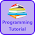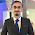# Programming Videos

## Search

### C++ Program to Print Even Numbers between 1 to 100 using For & While Loop

• Write a C++ program to print even numbers between 1 to 100.
• Write a C++ program to print even numbers between 1 to N.

In this tutorial, we are going to write a c++ code which print even numbers between 1 to 100 using for and while loop.

C program to print even numbers between 1 to 100

## C++ Program to Print Even Numbers between 1 to 100 using For Loop

```#include <iostream>
using namespace std;

int main() {

int i;

/* Run a loop from 1 to 100. */

for(i = 1; i < = 100; i++){

/* If number is divisible by 2. */

if(i % 2 == 0) {

cout << i <<" ";
}
}
return 0;
}

```

Output :

2 4 6 8 10 12 14 16 18 20 22 24 26 28 30 32 34 36 38 40
42 44 46 48 50 52 54 56 58 60 62 64 66 68 70
72 74 76 78 80 82 84 86 88 90 92 94 96 98 100

## C++ Program to Print Even numbers between 1 to 100 using While Loop

```#include <iostream>
using namespace std;

int main() {

/* Initialize i with 1. */

int i=1;

/* If i is less than or equal to 100. */

while( i <= 100){

/* If number is divisible by 2, then print.*/

if(i % 2 == 0){

cout <<i<< " ";
}

/* Increment i. */

i++;
}

return 0;
}

```

## C++ Program to Print Even numbers between 1 to N

In above examples, we have written a code to print even numbers between 1 to 100 using for and while loop. In this example, instead of 100 we take a input value from user and print even numbers between 1 to n (where n is input by user).

```#include <iostream>
using namespace std;

int main() {

/* Initialize i with 1. */

int i=1, n;

cout << "Enter a number \n";
cin >> n;

/* If i is less than or equal to n. */

while( i <= n){

/* If number is divisible by 2
then it's an even number
*/

if(i % 2 == 0){

cout <<i<< " ";
}

/* Increment i. */

i++;
}

return 0;
}

```

Programming question on strings

Sorting algorithm

1.2.3.